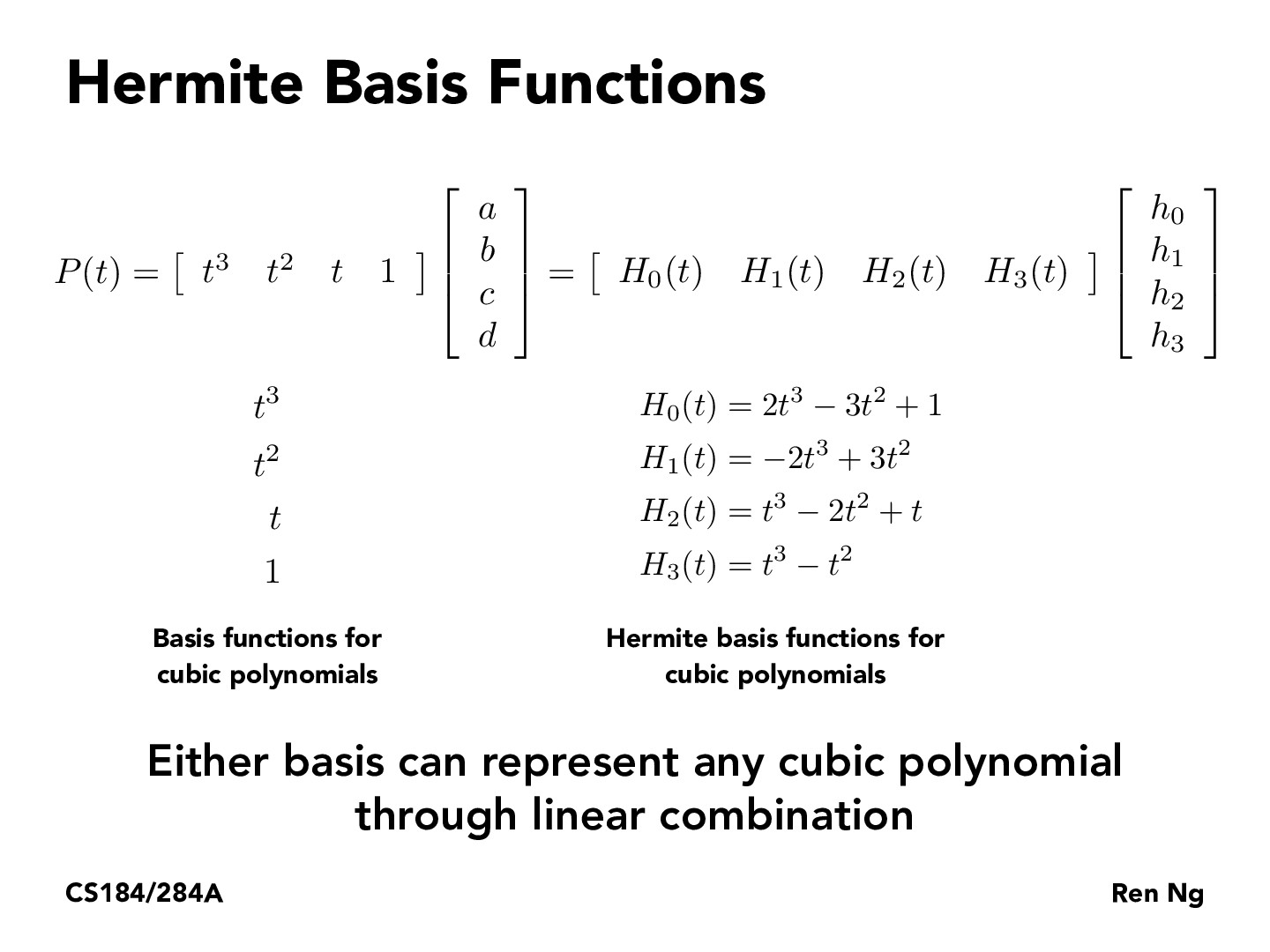Lecture 7: Geometry and Curves (36)lawrenceyan

For anyone that was confused as to where the values for the polynomials in the Hermite basis functions are coming from, the equations shown are simply summarizing the steps we took in the previous slides. So H_0(t), H_1(t), ... are just the values that we found by solving for the linear system we created originally using P(t) = at^3 + bt^2 + ct + d and P'(t) = 3at^2 + 2bt + c

You must be enrolled in the course to comment